# Rig Engine Power Consumption and Engine Efficiency

In this article, we will focus on the rig engine power consumption and overall engine efficiency and there are few examples for you to get more understand on how to use the formulas as well.Input Power of Engine

The formula is listed below;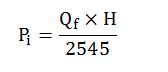Where;

Pi = input power in horse power (hp)

H = fuel heating value in BTU/lb

Qf = fuel consumption rate in lbm/hr

Fuel Consumption

The formula is listed below;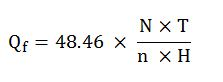Where;

Qf = fuel consumption rate in lb/hr

N = rotary speed of engine in revolution per minute, rpm

T = output torque, ft-lb

n = engine efficiency

H = fuel heating value in BTU/lb

Output Power of Engine

The formula is listed below;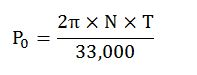Where;

N = rotary speed of engine in revolution per minute, rpm

T = output torque, ft-lb

Engine Efficiency

The formula is listed below;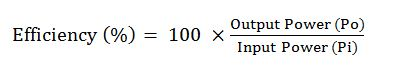Example#1: Rig engine runs at 1,600 rpm and the average output torque is 1,900 ft-lb. The engine consumes fuel about 200 lb mass per hour. Heating value of the fuel is 20,000 BTU/lb.

From the given information, determine the following values;

• Input Power (Pi)
• Output Power (Po)
• Engine Efficiency

Input Power (Pi)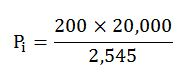Pi = 1572 hp

Output Power (Po)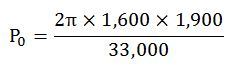Po = 579 hp

Engine Efficiency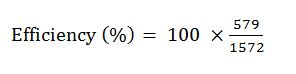Efficiency (%) =36.8 %

Example#2:  Diesel engine on the rig runs at 1,700 rpm and its output torque is 2,500 ft-lb. The average engine efficiency is about 35%.

Diesel fuel data: Heating value = 20,000 BTU/lb. Price per gallon = 1.1 \$/gal. Weight = 7.15 lb/gal.

Determine daily fuel cost from the rig diesel engine based on the given information.

Output Power (Po)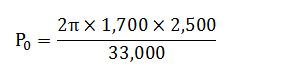Po = 809 hp

Input Power (Pi) based on the engine efficiency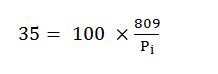Pi = 2,311 hp

Based on the input power formula, we can determine fuel rate consumption.Re-arrange the equation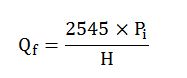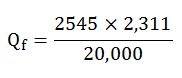Qf = 292 lbm/hr

Convert Qf from lbm/hr to gal/day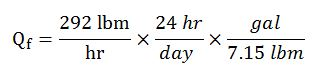Qf = 980 gal/day

Total fuel cost = Qf x Fuel price

Total fuel cost = 980 x 1.1 =1078 \$/day

Ref books:

Lapeyrouse, N.J., 2002. Formulas and calculations for drilling, production and workover, Boston: Gulf Professional publishing.

Bourgoyne, A.J.T., Chenevert , M.E. & Millheim, K.K., 1986. SPE Textbook Series, Volume 2: Applied Drilling Engineering, Society of Petroleum Engineers.

Mitchell, R.F., Miska, S. & Aadny, B.S., 2011. Fundamentals of drilling engineering, Richardson, TX: Society of Petroleum Engineers.

Share the joyWorking in the oil field and loving to share knowledge.

### 2 Responses to Rig Engine Power Consumption and Engine Efficiency

1.HERMINIO NAVARRO says:

In fuel consumption :

Qf = 48.46 ( N x T)/n x H
How does 48.46 has been derived

•DrillingFormulas.Com says:

This is the conversion factor. We will share it later.

This site uses Akismet to reduce spam. Learn how your comment data is processed.